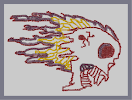### Flaming SkullHover over the thumbnail for a full-size version.

Author metallica_rulz author:metallica_rulz n-art rated 2009-04-04 2009-04-04 4 by 9 people. \$Flaming Skull#metallica_rulz#none#00000000000000000000000000000000000000000000000000000000000000000000000000000000000000000000000000000000000000000000000000000000000000000000000000000000000000000000000000000000000000000000000000000000000000000000000000000000000000000000000000000000000000000000000000000000000000000000000000000000000000000000000000000000000000000000000000000000000000000000000000000000000000000000000000000000000000000000000000000000000000000000000000000000000000000000000000000000000000000000000000000000000000000000000000000000000000000000000000000000000000000000000000000000000000000000000000000000000000000000000000000000000000000000000000000000000000000000000000000000000000000000000000000000000000000000000000000000000000000|12^718,316!12^713,322!12^710,327!12^711,333!12^710,338!12^709,345!12^703,351!12^697,353!12^691,353!12^686,353!12^683,357!12^683,364!12^684,373!12^685,377!12^689,381!12^688,387!12^682,388!12^675,395!12^678,395!12^669,399!12^665,405!12^660,408!12^652,405!12^649,405!12^651,411!12^658,415!12^655,420!12^650,420!12^647,426!12^647,432!12^648,440!12^647,444!12^649,451!12^649,458!12^650,462!12^644,470!12^642,476!12^640,481!12^634,486!12^631,486!12^631,483!12^631,479!12^632,475!12^632,468!12^627,458!12^622,466!12^621,472!12^617,473!12^614,476!12^612,480!12^607,486!12^600,492!12^597,500!12^590,510!12^549,513!12^536,516!12^522,517!12^509,521!12^497,527!12^490,532!12^484,533!12^475,535!12^464,537!12^454,537!12^448,539!12^440,540!12^439,539!12^404,542!12^395,543!12^390,542!12^381,544!12^376,542!12^377,535!12^412,538!12^442,501!12^445,497!12^429,498!12^419,499!12^417,497!12^425,491!12^430,487!12^433,483!12^435,476!12^434,472!12^436,463!12^439,451!12^440,443!12^440,437!12^435,436!12^425,431!12^414,429!12^409,428!12^394,426!12^370,420!12^374,397!12^344,390!12^332,392!12^325,392!12^315,393!12^293,393!12^286,392!12^280,390!12^274,390!12^271,387!12^273,387!12^278,386!12^286,385!12^295,381!12^301,379!12^309,378!12^316,376!12^321,374!12^308,372!12^294,372!12^279,373!12^264,374!12^251,376!12^243,376!12^233,376!12^225,376!12^212,376!12^203,376!12^195,376!12^170,379!12^157,376!12^155,375!12^151,369!12^155,366!12^159,364!12^164,356!12^170,352!12^177,350!12^186,344!12^198,342!12^205,342!12^214,342!12^223,340!12^231,335!12^242,330!12^236,330!12^229,330!12^219,330!12^212,330!12^204,331!12^200,334!12^197,336!12^248,344!12^256,344!12^266,342!12^276,338!12^279,334!12^281,332!12^278,328!12^266,328!12^258,331!12^251,336!12^177,336!12^165,336!12^158,336!12^154,336!12^151,332!12^144,328!12^134,324!12^121,327!12^111,327!12^101,324!12^93,320!12^93,315!12^101,310!12^116,308!12^130,308!12^141,308!12^148,308!12^157,303!12^170,298!12^175,297!12^180,295!12^186,294!12^190,293!12^199,290!12^207,290!12^214,290!12^225,296!12^231,298!12^236,296!12^247,294!12^251,293!12^257,292!12^264,292!12^280,288!12^293,283!12^301,283!12^310,280!12^317,272!12^309,271!12^289,271!12^220,266!12^174,273!12^168,272!12^158,271!12^144,269!12^140,269!12^133,266!12^128,261!12^120,258!12^110,254!12^101,250!12^85,246!12^64,244!12^52,244!12^48,245!12^51,239!12^57,235!12^66,232!12^74,230!12^79,230!12^83,237!12^135,238!12^127,236!12^124,232!12^121,228!12^129,228!12^139,228!12^148,228!12^161,230!12^170,230!12^183,235!12^195,235!12^203,235!12^211,235!12^215,232!12^207,228!12^201,221!12^197,215!12^201,218!12^204,216!12^212,216!12^218,216!12^228,216!12^235,225!12^239,225!12^243,224!12^253,224!12^259,224!12^268,225!12^276,225!12^281,225!12^288,225!12^308,225!12^312,225!12^303,223!12^291,218!12^282,218!12^273,218!12^281,214!12^291,211!12^299,209!12^306,207!12^316,208!12^321,209!12^330,210!12^343,216!12^354,216!12^361,213!12^376,209!12^376,203!12^367,201!12^357,200!12^333,200!12^318,200!12^306,198!12^293,197!12^284,200!12^274,203!12^271,203!12^256,206!12^249,203!12^242,200!12^237,196!12^238,194!12^248,192!12^261,191!12^270,191!12^279,190!12^280,186!12^271,185!12^260,180!12^253,175!12^246,173!12^236,173!12^223,175!12^212,177!12^193,181!12^184,181!12^184,166!12^176,166!12^161,164!12^149,162!12^145,158!12^145,155!12^152,152!12^166,152!12^178,154!12^185,157!12^192,160!12^200,160!12^212,160!12^220,160!12^234,166!12^241,166!12^248,166!12^260,164!12^265,161!12^275,154!12^276,158!12^283,162!12^293,163!12^304,165!12^320,163!12^324,168!12^355,163!12^356,160!12^347,155!12^335,151!12^324,146!12^314,140!12^301,135!12^294,122!12^284,121!12^269,120!12^258,120!12^242,126!12^228,130!12^215,128!12^205,126!12^195,126!12^172,126!12^156,126!12^149,127!12^139,126!12^131,125!12^122,125!12^103,125!12^92,125!12^86,125!12^79,125!12^77,124!12^86,119!12^91,117!12^99,115!12^104,111!12^96,111!12^91,110!12^82,107!12^75,110!12^71,111!12^65,113!12^62,110!12^65,106!12^71,102!12^76,101!12^87,97!12^95,95!12^105,95!12^113,95!12^121,95!12^134,95!12^144,95!12^160,96!12^166,102!12^174,102!12^180,100!12^195,97!12^213,98!12^223,98!12^230,103!12^249,102!12^248,97!12^244,94!12^234,91!12^221,91!12^214,91!12^204,91!12^197,90!12^191,85!12^197,79!12^208,78!12^217,80!12^237,82!12^245,82!12^247,82!12^254,81!12^266,82!12^276,86!12^287,89!12^304,95!12^310,96!12^308,87!12^300,81!12^297,78!12^283,76!12^287,74!12^296,75!12^315,80!12^324,80!12^331,84!12^343,91!12^347,93!12^354,96!12^361,98!12^386,112!12^379,107!12^379,106!12^373,103!12^365,98!12^391,115!12^391,108!12^388,102!12^383,94!12^367,81!12^382,88!12^394,92!12^401,93!12^407,95!12^420,96!12^426,100!12^435,102!12^443,107!12^448,109!12^451,102!12^438,95!12^438,95!12^440,94!12^446,94!12^453,95!12^464,99!12^472,100!12^481,100!12^522,116!12^517,116!12^514,114!12^506,108!12^498,104!12^493,102!12^487,102!12^575,149!12^573,147!12^570,140!12^565,136!12^558,130!12^548,126!12^539,124!12^530,120!12^523,118!12^572,143!12^566,136!12^565,129!12^566,124!12^580,128!12^582,130!12^587,135!12^591,137!12^602,139!12^611,149!12^619,157!12^624,166!12^631,176!12^634,181!12^633,167!12^633,164!12^633,158!12^633,154!12^638,151!12^646,155!12^661,166!12^710,237!12^707,233!12^702,226!12^699,221!12^697,216!12^695,210!12^691,206!12^687,199!12^683,191!12^676,183!12^670,175!12^664,169!12^656,162!12^647,156!12^693,237!12^687,231!12^684,225!12^674,218!12^669,214!12^663,209!12^658,206!12^652,202!12^640,194!12^634,189!12^630,186!12^625,185!12^615,180!12^609,175!12^598,171!12^586,167!12^574,164!12^567,164!12^554,164!12^536,165!12^515,166!12^505,169!12^496,172!12^486,171!12^478,170!12^471,169!12^460,169!12^450,170!12^452,170!12^458,173!12^467,177!12^450,177!12^443,178!12^427,178!12^417,177!12^408,182!12^415,182!12^424,182!12^436,182!12^446,184!12^433,199!12^422,201!12^408,203!12^393,206!12^379,213!12^375,220!12^389,220!12^398,220!12^414,220!12^416,227!12^421,232!12^432,232!12^439,250!12^429,253!12^410,255!12^395,259!12^386,259!12^378,262!12^365,278!12^352,278!12^352,278!12^355,291!12^360,291!12^370,291!12^388,291!12^406,289!12^423,303!12^432,307!12^433,311!12^433,311!12^416,325!12^416,325!12^386,328!12^384,328!12^371,328!12^364,329!12^393,324!12^405,322!12^416,322!12^429,322!12^436,314!12^444,311!12^360,330!12^354,333!12^353,336!12^359,343!12^363,343!12^373,343!12^379,343!12^386,343!12^399,343!12^407,341!12^452,337!12^467,347!12^472,349!12^466,357!12^458,360!12^450,361!12^442,361!12^424,362!12^422,368!12^424,374!12^424,385!12^424,399!12^425,419!12^426,426!12^719,318!12^720,317!12^720,314!12^720,307!12^720,301!12^720,294!12^720,283!12^719,274!12^717,266!12^715,261!12^711,248!12^647,333!12^657,333!12^661,333!12^667,333!12^674,333!12^676,331!12^679,326!12^681,321!12^682,319!12^683,313!12^685,309!12^685,308!12^685,301!12^685,299!12^684,295!12^679,287!12^677,284!12^674,282!12^665,278!12^660,276!12^654,276!12^648,276!12^642,276!12^637,276!12^632,276!12^626,280!12^624,283!12^623,291!12^622,296!12^622,303!12^624,305!12^627,310!12^627,311!12^628,317!12^630,319!12^636,327!12^639,332!12^643,332!12^672,336!12^670,336!12^665,336!12^652,337!12^648,337!12^643,337!12^638,337!12^632,337!12^625,337!12^620,332!12^616,326!12^615,319!12^615,311!12^615,303!12^616,296!12^617,290!12^627,280!12^627,280!12^627,294!12^627,304!12^621,289!12^621,289!12^615,295!12^612,304!12^610,312!12^609,321!12^609,325!12^609,332!12^609,337!12^613,341!12^618,343!12^634,344!12^638,343!12^643,342!12^649,340!12^659,338!12^657,336!12^638,336!12^635,336!12^630,332!12^625,325!12^621,310!12^620,314!12^618,330!12^618,337!12^626,341!12^626,341!12^626,326!12^623,319!12^619,326!12^629,329!12^646,327!12^657,327!12^664,326!12^667,318!12^673,309!12^676,300!12^675,292!12^658,283!12^635,286!12^638,305!12^656,307!12^653,296!12^645,305!12^653,311!12^645,322!12^639,320!12^644,306!12^645,316!12^659,320!12^670,325!12^676,318!12^676,314!12^681,309!12^681,305!12^680,300!12^678,293!12^669,284!12^659,280!12^649,280!12^640,281!12^632,288!12^639,306!12^661,301!12^655,297!12^659,298!12^669,296!12^658,292!12^647,292!12^647,292!12^646,292!12^642,293!12^639,304!12^637,306!12^640,313!12^644,316!12^647,318!12^649,316!12^653,312!12^655,307!12^663,304!12^668,306!12^671,310!12^670,315!12^646,315!12^635,309!12^636,308!12^632,301!12^632,298!12^640,294!12^637,290!12^642,285!12^645,284!12^647,283!12^652,283!12^655,285!12^660,294!12^663,296!12^655,311!12^655,322!12^652,329!12^670,331!12^677,326!12^679,315!12^679,309!12^674,306!12^672,303!12^670,293!12^665,287!12^653,295!12^646,300!12^656,300!12^657,310!12^659,315!12^640,324!12^628,327!12^628,319!12^636,312!12^636,305!12^637,317!12^638,321!12^632,329!12^666,312!12^666,312!12^660,312!12^660,318!12^666,324!12^678,318!12^672,318!12^642,300!12^642,300!12^636,294!12^630,288!12^630,288!12^630,288!12^630,282!12^642,282!12^648,288!12^654,288!12^672,282!12^660,282!12^660,288!12^672,288!12^678,294!12^660,300!12^648,300!12^648,306!12^648,312!12^648,312!12^636,318!12^624,318!12^624,318!12^624,312!12^624,306!12^612,306!12^612,324!12^612,330!12^618,336!12^636,330!12^642,318!12^630,306!12^630,306!12^636,294!12^630,294!12^648,294!12^672,294!12^660,294!12^660,312!12^654,318!12^648,318!12^636,330!12^624,342!12^618,342!12^618,330!12^618,324!12^618,312!12^636,306!12^636,300!12^636,300!12^636,288!12^636,282!12^648,282!12^672,282!12^660,288!12^660,294!12^660,306!12^660,324!12^642,330!12^630,336!12^624,342!12^612,336!12^612,330!12^612,318!12^612,318!12^624,306!12^630,300!12^630,300!12^630,330!12^624,330!12^624,312!12^654,312!12^660,330!12^672,324!12^672,318!12^672,306!12^672,294!12^666,288!12^648,300!12^636,312!12^624,312!12^624,324!12^624,324!12^618,318!12^618,312!12^624,300!12^624,300!12^630,288!12^624,300!12^624,312!12^642,324!12^654,330!12^642,330!12^630,330!12^648,324!12^660,318!12^666,306!12^666,300!12^678,294!12^654,282!12^642,306!12^630,312!12^630,318!12^630,306!12^618,300!12^618,306!12^624,282!12^642,282!12^642,294!12^666,294!12^666,288!12^684,294!12^666,288!12^666,282!12^654,306!12^642,312!12^630,318!12^624,324!12^636,324!12^666,318!12^660,318!12^666,324!12^684,306!12^672,300!12^654,300!12^654,300!12^642,294!12^630,288!12^624,288!12^612,300!12^630,324!12^641,289!12^653,325!12^665,329!12^660,334!12^655,334!12^661,333!12^665,331!12^668,331!12^677,328!12^677,328!12^617,317!12^613,317!12^610,317!12^607,317!12^631,345!12^642,342!12^672,339!12^668,339!12^661,341!12^659,342!12^652,343!12^647,343!12^641,343!12^634,343!12^673,308!12^623,335!12^540,516!12^540,516!12^534,516!12^534,516!12^528,516!12^522,516!12^516,516!12^516,522!12^510,528!12^504,528!12^498,528!12^492,534!12^480,540!12^474,540!12^468,540!12^462,540!12^456,540!12^450,540!12^444,540!12^444,540!12^438,540!12^432,540!12^426,540!12^426,540!12^420,540!12^414,540!12^408,540!12^402,540!12^396,540!12^390,540!12^390,540!12^384,540!12^384,540!12^378,534!12^378,534!12^378,528!12^378,522!12^384,522!12^390,522!12^390,522!12^396,516!12^402,516!12^402,516!12^409,516!12^411,515!12^415,513!12^418,512!12^420,510!12^426,509!12^427,509!12^431,507!12^433,507!12^437,506!12^443,502!12^434,502!12^428,502!12^422,502!12^419,502!12^419,495!12^420,492!12^425,489!12^429,486!12^429,484!12^430,479!12^430,476!12^431,473!12^429,472!12^428,470!12^432,464!12^434,458!12^434,456!12^435,452!12^436,448!12^437,445!12^437,440!12^432,437!12^429,432!12^428,427!12^428,426!12^428,422!12^428,416!12^428,411!12^428,401!12^428,396!12^428,392!12^427,388!12^426,383!12^426,377!12^426,374!12^425,370!12^426,364!12^430,362!12^435,362!12^441,362!12^447,362!12^451,361!12^459,358!12^460,358!12^463,356!12^465,353!12^466,350!12^460,340!12^455,336!12^446,336!12^444,336!12^439,337!12^432,337!12^426,337!12^419,337!12^417,337!12^410,337!12^408,339!12^403,339!12^400,339!12^391,341!12^385,341!12^382,341!12^378,341!12^368,341!12^363,341!12^353,337!12^355,334!12^359,333!12^363,332!12^370,330!12^375,330!12^385,330!12^386,330!12^394,327!12^400,325!12^405,325!12^412,325!12^420,325!12^423,324!12^428,322!12^438,315!12^438,312!12^430,310!12^425,309!12^422,303!12^412,294!12^407,289!12^395,290!12^390,290!12^385,290!12^379,290!12^372,290!12^422,304!12^419,301!12^415,294!12^417,290!12^359,290!12^359,287!12^362,281!12^364,279!12^362,277!12^361,274!12^359,276!12^358,274!12^359,273!12^359,272!12^364,267!12^369,264!12^372,262!12^376,261!12^379,260!12^383,259!12^388,259!12^391,259!12^395,258!12^394,258!12^398,258!12^400,258!12^404,256!12^407,256!12^410,256!12^416,254!12^418,253!12^426,250!12^426,250!12^432,249!12^434,248!12^437,247!12^439,237!12^439,233!12^436,233!12^430,233!12^424,233!12^417,230!12^409,222!12^404,222!12^401,222!12^398,222!12^393,222!12^388,222!12^384,222!12^381,222!12^380,222!12^379,217!12^379,214!12^379,212!12^376,209!12^369,203!12^364,200!12^359,200!12^356,200!12^353,200!12^348,200!12^342,200!12^334,200!12^328,200!12^324,200!12^316,196!12^311,196!12^308,196!12^300,195!12^289,195!12^285,195!12^282,197!12^278,201!12^276,201!12^269,202!12^266,202!12^261,202!12^257,202!12^255,202!12^252,202!12^245,198!12^240,194!12^236,193!12^238,192!12^244,192!12^253,192!12^258,192!12^266,192!12^266,192!12^269,186!12^276,186!12^273,186!12^266,183!12^260,179!12^255,177!12^246,175!12^242,175!12^225,175!12^220,175!12^215,176!12^212,176!12^205,177!12^195,178!12^178,178!12^176,167!12^156,165!12^150,162!12^147,158!12^148,154!12^153,152!12^158,152!12^165,152!12^169,152!12^174,152!12^178,157!12^183,157!12^186,158!12^195,158!12^201,158!12^208,158!12^214,158!12^220,158!12^229,162!12^234,163!12^241,163!12^247,163!12^251,163!12^254,163!12^259,161!12^270,159!12^229,174!12^227,176!12^204,181!12^199,181!12^162,167!12^165,167!12^170,167!12^172,167!12^180,167!12^176,167!12^169,167!12^158,172!12^158,172!12^153,172!12^147,173!12^139,175!12^139,175!12^143,175!12^145,175!12^153,175!12^155,176!12^158,176!12^160,177!12^169,181!12^172,181!12^175,181!12^183,181!12^278,160!12^282,160!12^283,161!12^290,163!12^295,163!12^298,163!12^302,163!12^303,163!12^321,163!12^327,164!12^332,164!12^336,164!12^338,164!12^342,163!12^348,163!12^355,162!12^355,162!12^354,151!12^350,148!12^343,148!12^338,148!12^331,148!12^323,144!12^319,142!12^311,139!12^305,138!12^303,137!12^301,133!12^301,133!12^300,128!12^296,124!12^289,124!12^282,124!12^273,124!12^269,124!12^261,124!12^254,124!12^251,124!12^245,124!12^239,125!12^236,125!12^230,126!12^226,126!12^223,126!12^222,126!12^215,126!12^211,123!12^205,123!12^202,123!12^195,123!12^187,123!12^183,123!12^176,123!12^169,123!12^161,125!12^157,125!12^153,125!12^147,125!12^140,125!12^137,125!12^133,125!12^128,125!12^125,125!12^117,125!12^113,125!12^110,125!12^107,125!12^100,125!12^97,125!12^90,125!12^86,125!12^82,125!12^81,125!12^82,122!12^87,118!12^92,116!12^99,112!12^99,111!12^92,111!12^85,108!12^81,108!12^72,108!12^65,108!12^65,108!12^67,101!12^74,95!12^81,95!12^85,95!12^89,95!12^94,95!12^102,95!12^107,95!12^110,95!12^116,95!12^122,95!12^126,95!12^128,95!12^134,95!12^142,95!12^145,95!12^149,95!12^153,95!12^157,95!12^159,99!12^166,99!12^178,99!12^186,99!12^192,99!12^198,99!12^204,99!12^210,99!12^215,99!12^221,102!12^223,102!12^228,103!12^234,104!12^241,104!12^247,104!12^247,101!12^247,97!12^237,94!12^222,89!12^220,89!12^216,89!12^209,89!12^202,89!12^195,89!12^193,83!12^193,83!12^198,79!12^203,78!12^209,78!12^215,78!12^219,78!12^223,78!12^232,81!12^238,81!12^244,81!12^255,81!12^255,81!12^263,81!12^266,81!12^269,81!12^275,84!12^277,86!12^281,86!12^286,88!12^292,88!12^296,91!12^297,91!12^300,91!12^305,91!12^305,89!12^305,88!12^305,84!12^301,80!12^294,75!12^286,72!12^281,72!12^289,70!12^292,70!12^300,74!12^301,75!12^304,78!12^308,80!12^315,80!12^323,82!12^323,82!12^331,85!12^338,87!12^342,88!12^345,90!12^352,93!12^353,93!12^361,99!12^367,102!12^371,104!12^379,106!12^384,108!12^388,109!12^391,110!12^386,102!12^386,99!12^385,96!12^385,92!12^381,87!12^375,83!12^370,80!12^379,85!12^379,85!12^391,89!12^395,90!12^400,90!12^410,90!12^412,93!12^417,94!12^421,96!12^426,98!12^431,101!12^435,103!12^440,105!12^448,106!12^452,105!12^452,99!12^462,94!12^467,95!12^472,98!12^472,98!12^480,100!12^486,100!12^492,105!12^494,106!12^501,108!12^513,114!12^517,117!12^521,122!12^529,122!12^538,122!12^540,123!12^544,125!12^552,128!12^558,131!12^561,133!12^566,128!12^567,127!12^572,124!12^578,127!12^586,133!12^591,136!12^596,138!12^604,142!12^609,146!12^609,147!12^615,151!12^617,153!12^618,153!12^619,158!12^621,163!12^623,164!12^628,167!12^629,169!12^629,176!12^722,294!12^721,290!12^720,282!12^717,275!12^715,267!12^714,261!12^711,256!12^705,232!12^704,232!12^700,226!12^698,221!12^696,218!12^693,213!12^689,208!12^687,204!12^682,198!12^675,189!12^669,182!12^667,178!12^664,173!12^659,169!12^654,164!12^651,161!12^643,155!12^681,186!12^710,249!12^709,246!12^706,238!12^703,246!12^695,243!12^689,237!12^685,232!12^680,228!12^676,222!12^670,217!12^666,213!12^663,210!12^659,208!12^653,206!12^651,205!12^647,200!12^643,195!12^639,193!12^633,190!12^627,188!12^622,186!12^616,183!12^613,181!12^609,177!12^602,173!12^598,171!12^595,170!12^591,170!12^587,170!12^582,168!12^577,165!12^572,165!12^567,165!12^558,165!12^554,165!12^551,165!12^548,165!12^545,165!12^542,165!12^538,165!12^533,165!12^526,165!12^526,165!12^520,165!12^559,164!12^411,179!12^413,179!12^416,177!12^418,177!12^420,177!12^429,177!12^435,177!12^440,177!12^445,177!12^455,174!12^456,171!12^460,167!12^464,167!12^470,167!12^474,167!12^479,171!12^484,172!12^486,172!12^495,172!12^499,171!12^507,168!12^514,167!12^409,180!12^412,180!12^419,180!12^421,180!12^428,181!12^432,181!12^442,188!12^446,187!12^447,187!12^452,184!12^453,183!12^459,182!12^440,195!12^433,199!12^428,199!12^423,200!12^416,202!12^411,203!12^405,204!12^404,204!12^398,205!12^396,206!12^390,206!12^422,430!12^414,430!12^407,429!12^400,427!12^389,426!12^387,426!12^381,424!12^372,419!12^368,419!12^372,407!12^360,417!12^357,415!12^352,415!12^346,415!12^343,415!12^340,415!12^342,410!12^349,409!12^353,409!12^358,409!12^362,409!12^369,409!12^373,397!12^367,397!12^362,395!12^357,394!12^351,393!12^372,421!12^377,421!12^347,391!12^338,391!12^331,392!12^295,396!12^286,393!12^284,384!12^290,384!12^294,383!12^301,378!12^305,377!12^314,375!12^320,392!12^317,392!12^296,396!12^298,396!12^303,396!12^306,396!12^313,396!12^309,371!12^301,371!12^293,371!12^287,371!12^281,371!12^277,371!12^269,371!12^264,375!12^262,376!12^257,376!12^250,376!12^247,376!12^241,376!12^234,376!12^229,376!12^224,376!12^218,376!12^215,376!12^208,376!12^204,376!12^199,376!12^193,376!12^185,378!12^179,378!12^175,378!12^171,378!12^167,378!12^166,378!12^159,374!12^159,374!12^159,366!12^159,366!12^160,362!12^162,359!12^168,355!12^174,354!12^186,351!12^183,351!12^183,351!12^186,346!12^188,345!12^194,345!12^198,345!12^202,345!12^208,345!12^212,345!12^217,345!12^222,344!12^225,340!12^228,336!12^234,333!12^234,333!12^237,331!12^237,331!12^227,330!12^218,330!12^213,330!12^205,330!12^200,330!12^198,331!12^191,338!12^188,338!12^182,338!12^178,336!12^169,335!12^162,335!12^160,335!12^157,335!12^154,335!12^149,330!12^143,326!12^137,326!12^133,326!12^129,326!12^125,328!12^118,328!12^114,327!12^106,324!12^101,324!12^99,323!12^94,315!12^99,315!12^106,315!12^107,315!12^111,311!12^114,310!12^123,310!12^130,310!12^131,310!12^138,310!12^142,310!12^149,309!12^153,306!12^158,302!12^168,299!12^168,299!12^182,296!12^186,293!12^190,292!12^199,292!12^202,292!12^208,292!12^214,292!12^218,292!12^224,292!12^316,273!12^315,273!12^310,280!12^306,282!12^301,287!12^294,287!12^287,287!12^282,287!12^277,290!12^274,290!12^267,294!12^258,294!12^253,295!12^242,295!12^237,295!12^233,295!12^302,271!12^295,271!12^292,271!12^283,271!12^215,267!12^205,269!12^204,269!12^200,269!12^196,269!12^184,271!12^182,271!12^172,271!12^171,271!12^165,271!12^160,271!12^156,271!12^152,271!12^214,268!12^204,271!12^197,271!12^191,271!12^180,273!12^246,342!12^246,338!12^246,336!12^250,335!12^255,331!12^259,330!12^261,330!12^268,330!12^274,330!12^279,330!12^279,335!12^276,339!12^269,340!12^261,341!12^254,343!12^278,270!12^274,270!12^270,269!12^266,269!12^263,268!12^262,267!12^258,265!12^256,262!12^252,259!12^250,259!12^246,257!12^239,257!12^235,258!12^233,261!12^229,263!12^224,264!12^219,265!12^215,266!12^208,268!12^204,271!12^131,264!12^130,264!12^125,260!12^119,256!12^115,254!12^110,254!12^107,254!12^105,254!12^63,246!12^100,252!12^94,249!12^86,247!12^83,247!12^76,247!12^69,247!12^66,247!12^62,247!12^55,247!12^50,247!12^50,241!12^51,237!12^56,235!12^61,232!12^64,229!12^69,227!12^79,227!12^81,237!12^86,237!12^93,237!12^98,237!12^106,237!12^112,237!12^119,237!12^125,236!12^130,235!12^126,227!12^131,227!12^133,227!12^140,227!12^141,227!12^147,232!12^151,232!12^156,231!12^159,231!12^166,233!12^170,233!12^181,234!12^188,234!12^199,235!12^201,235!12^204,235!12^211,235!12^212,235!12^212,230!12^211,230!12^208,226!12^200,218!12^200,216!12^204,213!12^208,213!12^216,213!12^216,213!12^218,214!12^222,214!12^229,216!12^177,233!12^230,218!12^232,219!12^236,222!12^242,223!12^247,223!12^252,223!12^262,223!12^264,223!12^268,223!12^275,223!12^282,224!12^291,224!12^293,224!12^299,224!12^303,225!12^294,223!12^286,219!12^280,217!12^276,217!12^281,216!12^285,214!12^292,213!12^299,213!12^303,212!12^304,212!12^310,210!12^315,210!12^329,210!12^330,212!12^338,213!12^341,212!12^345,212!12^350,212!12^359,212!12^362,212!12^581,504!12^582,500!12^582,497!12^582,493!12^583,487!12^583,483!12^584,480!12^586,475!12^587,470!12^589,467!12^590,463!12^592,458!12^593,456!12^596,450!12^597,450!12^597,450!12^605,450!12^608,450!12^616,450!12^623,454!12^628,458!12^630,460!12^587,513!12^593,507!12^597,502!12^598,500!12^602,495!12^605,491!12^607,486!12^578,492!12^575,493!12^565,499!12^564,499!12^565,484!12^565,482!12^566,477!12^567,471!12^569,464!12^570,462!12^571,458!12^573,454!12^576,449!12^577,445!12^577,445!12^580,441!12^568,459!12^565,461!12^560,463!12^550,456!12^551,452!12^553,449!12^557,447!12^558,445!12^559,442!12^560,440!12^562,437!12^564,433!12^567,430!12^548,451!12^544,452!12^538,445!12^538,441!12^539,438!12^540,435!12^542,432!12^545,430!12^547,427!12^549,425!12^551,423!12^551,422!12^538,438!12^535,439!12^530,440!12^526,441!12^525,442!12^525,435!12^527,431!12^527,429!12^531,424!12^533,422!12^535,419!12^535,418!12^551,511!12^551,511!12^543,508!12^542,507!12^539,504!12^536,503!12^530,503!12^527,503!12^522,503!12^519,503!12^513,503!12^514,503!12^520,503!12^526,502!12^530,502!12^535,501!12^537,500!12^541,497!12^540,487!12^533,487!12^527,487!12^523,487!12^520,487!12^514,487!12^506,487!12^504,487!12^532,483!12^533,481!12^535,479!12^535,475!12^532,474!12^528,473!12^520,473!12^517,473!12^514,473!12^511,473!12^509,473!12^506,473!12^522,475!12^526,472!12^528,470!12^528,464!12^530,459!12^527,459!12^522,459!12^518,459!12^513,459!12^508,459!12^507,459!12^502,458!12^499,453!12^498,452!12^498,447!12^501,447!12^505,447!12^510,447!12^518,447!12^521,447!12^524,448!12^524,455!12^524,460!12^524,460!12^501,444!12^502,439!12^502,436!12^503,429!12^504,427!12^506,421!12^511,419!12^517,417!12^520,417!12^525,417!12^532,417!12^535,417!12^537,417!12^623,468!12^625,465!12^630,471!12^631,481!12^633,484!12^638,484!12^643,479!12^645,475!12^648,470!12^648,468!12^650,464!12^650,464!12^536,411!12^533,406!12^530,401!12^527,395!12^524,392!12^520,387!12^518,387!12^514,386!12^511,385!12^498,441!12^489,433!12^486,429!12^484,424!12^484,419!12^485,411!12^485,409!12^485,405!12^486,402!12^490,397!12^493,394!12^499,389!12^499,389!12^503,386!12^506,385!12^520,413!12^518,408!12^514,403!12^513,400!12^509,394!12^510,417!12^507,414!12^504,410!12^502,407!12^499,404!12^496,400!12^494,398!12^499,431!12^496,431!12^493,429!12^489,428!12^489,428!12^506,389!12^484,416!12^674,281!12^673,279!12^669,274!12^665,270!12^662,267!12^658,265!12^656,263!12^650,263!12^645,262!12^639,262!12^634,262!12^631,262!12^626,262!12^621,262!12^618,262!12^610,268!12^608,270!12^605,272!12^599,280!12^599,281!12^595,287!12^590,295!12^590,298!12^590,300!12^590,307!12^590,313!12^590,319!12^590,323!12^591,330!12^591,332!12^591,336!12^591,340!12^592,342!12^598,346!12^601,346!12^603,348!12^605,348!12^611,348!12^618,348!12^624,348!12^627,347!12^631,346!12^633,346!12^593,292!12^594,291!12^595,286!12^599,280!12^604,276!12^608,272!12^612,267!12^613,265!12^617,263!12^622,260!12^504,524!12^499,518!12^498,516!12^497,513!12^490,511!12^490,504!12^489,501!12^489,500!12^488,497!12^488,494!12^486,488!12^486,483!12^486,479!12^486,476!12^485,471!12^485,465!12^485,457!12^485,452!12^485,445!12^485,441!12^486,437!12^486,435!12^486,435!12^484,460!12^482,454!12^487,490!12^647,460!12^647,460!12^644,454!12^642,451!12^640,447!12^632,443!12^628,437!12^623,432!12^616,426!12^612,423!12^606,419!12^603,417!12^599,415!12^594,412!12^591,412!12^583,406!12^579,399!12^574,397!12^570,397!12^569,397!12^565,397!12^559,395!12^553,394!12^550,393!12^542,391!12^539,389!12^534,388!12^529,388!12^526,388!12^523,386!12^583,406!12^586,406!12^499,522!12^583,436!12^583,435!12^585,431!12^585,426!12^589,422!12^589,418!12^590,415!12^590,411!12^569,423!12^570,420!12^571,416!12^572,412!12^574,411!12^576,405!12^579,398!12^552,419!12^552,417!12^555,412!12^557,409!12^559,407!12^559,404!12^560,401!12^562,395!12^593,445!12^597,442!12^597,439!12^599,436!12^603,431!12^605,427!12^605,427!12^605,427!12^608,423!3^602,338!3^601,337!3^598,332!3^600,329!3^603,325!3^603,324!3^600,321!3^599,319!3^598,317!3^598,312!3^598,309!3^599,303!3^599,299!3^600,298!3^602,294!3^603,291!3^604,289!3^606,287!3^607,286!3^608,282!3^611,278!3^615,276!3^618,273!3^622,271!3^625,269!3^627,268!3^631,268!3^637,268!3^642,268!3^648,268!3^652,268!3^651,272!3^654,272!3^606,315!3^606,312!3^606,305!3^607,301!3^607,295!3^609,291!3^612,285!3^616,281!3^621,278!3^624,277!3^609,343!3^609,343!12^428,411!12^428,405!12^428,404!12^428,319!12^429,317!12^434,317!12^440,313!12^382,328!12^388,328!0^542,161!0^542,161!0^538,156!0^533,149!0^531,148!0^528,145!0^525,142!0^522,139!0^518,135!0^515,130!0^512,127!0^508,122!0^505,120!0^500,118!0^497,118!0^491,118!0^490,118!0^486,118!0^485,118!0^485,119!0^486,121!0^488,124!0^489,125!0^490,127!0^491,131!0^491,132!0^491,134!0^491,134!0^485,134!0^481,133!0^478,133!0^474,130!0^469,126!0^462,123!0^459,121!0^455,119!0^453,119!0^449,118!0^443,118!0^436,114!0^433,114!0^429,114!0^427,114!0^423,114!0^423,114!0^424,118!0^425,119!0^428,121!0^430,123!0^431,124!0^432,127!0^436,131!0^437,131!0^439,135!0^437,135!0^432,135!0^426,135!0^425,135!0^421,135!0^418,135!0^417,135!0^408,133!0^405,132!0^405,132!0^402,131!0^398,128!0^396,128!0^394,127!0^385,123!0^383,122!0^380,121!0^378,119!0^373,116!0^370,115!0^368,115!0^360,113!0^359,113!0^355,114!0^350,116!0^349,116!0^347,114!0^345,114!0^336,107!0^331,104!0^331,104!0^328,104!0^327,104!0^321,105!0^315,107!0^314,108!0^306,108!0^306,108!0^343,112!0^341,112!0^336,108!0^333,104!0^309,112!0^312,112!0^316,117!0^316,118!0^318,120!0^319,122!0^324,124!0^328,124!0^333,124!0^341,125!0^344,125!0^349,125!0^353,128!0^353,130!0^353,133!0^354,136!0^354,138!0^360,143!0^365,143!0^368,142!0^372,141!0^375,141!0^379,142!0^379,144!0^379,149!0^379,154!0^379,157!0^379,160!0^374,164!0^371,167!0^368,169!0^363,172!0^358,171!12^324,166!12^317,166!12^313,166!12^309,166!12^307,166!12^305,166!0^356,171!0^353,171!0^349,171!0^343,173!0^340,174!0^332,179!0^332,179!0^334,179!0^340,180!0^341,180!0^344,181!0^346,183!0^348,183!0^352,184!0^353,185!0^359,186!0^360,186!0^364,187!0^367,190!0^371,197!0^373,197!0^374,199!0^376,205!0^378,206!0^379,206!12^387,208!12^385,208!12^383,208!12^378,208!12^376,207!12^373,204!12^370,201!0^377,221!0^377,221!0^370,224!0^368,225!0^366,225!0^363,227!0^359,231!0^356,233!0^352,233!0^347,233!0^343,232!0^339,230!0^335,227!0^331,224!0^331,224!0^329,226!0^328,227!0^322,232!0^320,235!0^317,238!0^309,242!0^305,243!0^303,243!0^301,243!0^298,243!0^297,243!0^294,242!0^287,241!0^282,239!0^278,237!0^276,237!0^272,237!0^270,237!0^266,237!0^262,237!0^258,236!0^255,236!0^249,236!0^244,236!0^236,236!0^232,238!0^228,240!0^227,241!0^227,242!0^231,242!0^236,242!0^239,242!0^241,242!0^246,244!0^248,244!0^252,244!0^252,248!0^253,248!0^255,250!0^256,252!0^258,253!0^259,253!0^262,253!0^266,253!0^272,254!0^276,256!0^280,256!0^287,256!0^290,256!0^292,256!0^300,256!0^305,258!0^308,259!0^318,259!0^320,259!0^324,259!0^327,257!0^330,254!0^339,250!0^339,250!0^314,259!0^333,254!0^341,254!0^343,254!0^341,259!0^341,264!0^340,268!0^339,275!0^339,279!0^339,280!0^338,288!0^338,292!0^338,295!0^337,297!0^334,298!0^331,300!0^329,301!0^325,301!0^322,301!0^316,301!0^310,297!0^309,296!0^304,295!0^300,295!0^292,297!0^290,298!0^287,299!0^283,303!0^277,307!0^275,308!0^272,309!0^267,312!0^265,312!0^259,312!0^254,311!0^250,311!0^239,311!0^236,309!0^243,313!0^236,309!0^229,306!0^225,306!0^221,306!0^217,306!0^214,306!0^213,307!0^213,311!0^213,316!0^215,316!0^217,316!0^220,316!0^224,316!0^224,316!0^228,317!0^232,317!0^234,317!0^235,317!0^239,317!0^241,317!0^243,317!0^245,317!0^250,317!0^252,317!0^256,317!0^259,317!0^263,317!0^268,317!0^272,317!0^274,320!0^275,320!0^276,321!0^281,325!0^284,325!0^286,325!0^286,325!0^282,325!0^277,323!0^291,325!0^297,322!0^305,320!0^306,320!0^308,320!0^314,320!0^317,320!0^322,320!0^323,320!0^323,325!0^323,329!0^323,332!0^322,333!0^320,334!0^315,336!0^312,338!0^309,340!0^305,340!0^302,340!0^300,341!0^297,342!0^293,345!0^292,345!0^290,345!0^286,345!0^282,346!0^276,347!0^275,347!0^272,349!0^271,350!0^268,350!0^266,350!0^263,350!0^257,350!0^257,350!0^253,350!0^251,350!0^247,350!0^243,350!0^239,350!0^239,350!0^239,351!0^237,352!0^236,353!0^235,355!0^231,355!0^231,356!0^229,357!0^229,361!0^231,361!0^235,361!0^238,361!0^241,361!0^245,363!0^249,363!0^251,363!0^259,362!0^259,360!0^261,359!0^262,359!0^265,359!0^272,356!0^272,356!0^278,356!0^280,356!0^281,356!0^281,356!0^285,357!0^286,357!0^289,357!0^295,357!0^303,357!0^307,356!0^312,356!0^315,355!0^316,354!0^319,352!0^321,350!0^325,350!0^325,350!0^327,349!0^328,349!0^333,347!0^335,347!0^339,347!0^342,347!0^343,347!0^344,347!0^346,350!0^346,351!0^346,352!0^346,352!0^346,353!0^346,355!0^345,356!0^344,358!0^342,358!0^342,359!0^338,364!0^336,365!0^334,367!0^332,368!0^332,371!0^332,374!0^332,374!0^334,374!0^341,374!0^343,374!0^347,372!0^351,372!0^354,372!0^356,371!0^360,370!0^363,368!0^363,367!0^363,366!0^364,364!0^367,361!0^367,361!0^367,362!0^365,366!0^364,368!0^362,369!0^359,372!0^358,373!0^353,377!0^353,377!0^353,377!0^357,377!0^359,377!0^362,378!0^362,378!0^364,378!0^365,378!0^367,379!0^368,379!0^372,384!0^376,384!0^380,382!0^381,381!0^383,380!0^386,375!0^389,372!0^392,372!0^396,371!0^399,371!0^399,373!0^399,374!0^399,378!0^414,387!0^411,415!0^413,415!0^416,415!0^420,415!12^377,408!12^378,408!12^379,407!12^381,406!12^384,404!12^386,404!12^389,404!12^391,403!12^392,402!12^390,402!12^388,402!12^383,400!12^379,398!12^376,397!12^375,397!12^373,397!0^399,382!0^398,383!0^398,384!0^398,385!0^394,389!0^392,390!0^389,392!0^386,393!0^384,395!0^385,395!0^391,395!0^394,393!0^396,392!0^399,391!0^400,391!0^404,390!0^406,390!0^410,389!0^413,388!0^415,387!0^416,387!0^416,387!0^416,387!0^416,387!0^414,389!0^412,393!0^410,396!0^410,396!0^406,399!0^405,401!0^400,405!0^398,406!0^395,407!0^394,408!0^394,411!0^395,411!0^397,411!0^397,411!0^400,411!0^404,414!0^411,416!0^407,414!0^393,125!0^392,125!0^387,124!0^375,221!0^375,221!0^379,218!0^379,216!0^379,216!0^379,213!0^379,210!0^379,209!0^379,209!0^378,207!0^378,205!0^378,203!0^378,203!12^565,497!12^565,495!12^565,492!12^565,490!12^566,485!12^569,502!12^570,498!12^573,497!12^577,492!12^545,519!12^550,514!12^549,510!12^545,508!12^541,506!12^543,496!12^544,495!12^545,493!12^546,490!12^546,490!12^546,487!12^543,487!12^539,487!10^491,424!10^493,424!10^498,424!10^500,421!10^502,415!10^502,411!10^501,406!10^497,403!10^495,400!10^492,396!10^491,394!10^490,393!10^490,390!10^496,386!10^502,386!10^503,390!10^507,391!10^508,394!10^512,398!10^514,402!10^517,406!10^519,410!10^519,410!10^522,410!10^527,410!10^530,410!10^533,410!10^536,410!10^536,409!10^536,409!10^537,404!10^537,402!10^536,399!10^534,398!10^531,395!10^528,392!10^528,392!10^526,389!10^532,389!10^535,389!10^538,389!10^540,389!10^544,389!10^546,389!10^551,390!10^556,392!10^558,393!10^558,399!10^558,403!10^556,406!10^554,408!10^551,408!10^551,408!10^549,409!10^543,410!10^537,410!10^532,410!10^525,388!10^520,388!10^514,388!10^509,387!10^485,420!10^485,417!10^485,414!10^483,410!10^484,403!10^484,399!12^511,503!12^511,503!12^507,503!12^503,503!12^503,503!12^500,503!12^497,503!12^498,486!12^497,486!12^494,486!12^491,486!12^489,486!12^505,473!12^502,473!12^500,473!12^496,473!12^493,473!12^489,473!12^486,473!12^486,473!12^496,462!12^496,462!12^492,464!12^488,465!12^501,462!12^501,462!0^518,162!0^518,161!0^516,159!0^513,156!0^511,153!0^509,150!0^505,146!0^503,144!0^500,141!0^493,134!0^491,131!0^490,129!0^492,163!0^490,160!0^485,155!0^482,152!0^479,146!0^477,144!0^473,138!0^470,135!0^468,133!0^464,130!0^462,128!0^461,126!0^461,125!0^456,121!0^490,163!0^475,165!0^472,161!0^465,156!0^459,150!0^454,146!0^452,144!0^447,141!0^445,139!0^443,139!0^440,137!0^439,135!0^436,134!0^428,129!0^443,171!0^443,171!0^439,168!0^434,164!0^433,163!0^431,161!0^428,157!0^423,153!0^422,151!0^420,149!0^417,146!0^414,145!0^410,144!0^406,142!0^402,142!0^399,142!0^396,142!0^393,142!0^390,142!0^385,142!0^380,157!0^381,157!0^384,157!0^384,157!0^387,157!0^388,160!0^388,160!0^391,162!0^392,163!0^394,163!0^401,163!0^405,163!0^407,163!0^412,165!0^414,166!0^415,172!0^411,180!0^408,179!0^402,179!0^400,179!0^398,179!0^392,179!0^389,179!0^385,179!0^380,179!0^376,179!0^371,179!0^367,179!0^362,180!0^359,180!0^358,181!0^500,142!0^499,142!0^496,136!0^390,221!0^390,224!0^389,227!0^382,228!0^379,229!0^375,232!0^370,233!0^365,233!0^362,232!0^362,232!0^357,230!0^357,230!12^440,240!12^438,241!12^431,246!12^424,247!12^415,252!12^413,291!12^410,288!12^404,288!12^398,287!12^394,287!12^388,288!12^380,288!12^376,288!12^369,289!12^369,289!12^366,289!12^365,289!0^411,250!0^401,250!0^398,250!0^394,250!0^390,250!0^387,249!0^383,246!0^381,244!0^377,244!0^374,244!0^370,244!0^365,244!0^358,247!0^355,249!0^352,252!0^348,253!0^348,253!0^360,281!0^358,281!0^355,278!0^354,275!0^351,273!0^349,273!0^346,274!0^342,276!0^339,276!0^369,291!0^367,292!0^364,292!0^360,294!0^357,295!0^354,296!0^349,298!0^346,300!0^343,303!0^342,308!0^341,311!0^339,314!0^339,313!0^335,315!0^333,316!0^331,316!0^326,317!0^335,340!0^335,336!0^336,334!0^339,329!0^340,325!0^346,319!0^350,318!0^354,318!0^357,318!0^359,317!0^363,315!0^367,315!0^368,314!0^373,312!0^380,310!0^384,309!0^387,307!0^398,302!0^401,299!0^403,299!0^412,292!0^394,305!0^396,303!0^403,299!0^405,298!0^410,295!0^369,361!0^370,359!0^374,357!0^379,351!0^381,350!0^385,346!0^387,344!0^387,344!0^393,374!0^397,367!0^397,367!0^400,365!0^402,364!0^403,361!0^409,354!0^409,354!0^409,352!0^412,348!0^414,346!0^415,343!0^416,341!0^407,383!0^408,379!0^408,376!0^409,375!0^410,372!0^412,368!0^415,366!0^417,363!0^417,362!0^425,359!0^425,358!0^426,356!0^427,355!0^429,353!0^435,347!0^435,347!0^439,342!0^443,340!0^441,340!0^433,350!0^410,402!0^412,402!0^413,402!0^416,402!0^420,402!0^421,402!0^421,402!3^696,228!3^690,216!3^684,210!3^678,203!3^670,190!3^662,178!3^651,167!3^643,160!3^641,167!3^639,182!3^639,177!3^624,179!3^618,171!3^613,163!3^610,160!3^603,151!3^600,148!3^596,143!3^590,137!3^587,136!3^585,136!3^583,135!3^580,133!3^568,130!3^569,131!3^571,138!3^572,142!3^573,146!3^571,148!3^568,148!3^564,143!3^562,138!3^561,136!3^555,132!3^551,130!3^546,130!3^537,128!3^535,128!3^529,124!3^525,124!3^520,123!3^506,116!3^506,116!3^506,115!3^502,111!3^500,111!3^500,111!3^495,109!3^492,108!3^489,107!3^485,106!3^480,105!3^479,104!3^475,102!3^471,102!3^467,102!3^463,101!3^453,96!3^453,102!3^454,104!3^448,104!3^447,104!3^442,105!3^438,105!3^437,104!3^433,101!3^430,100!3^425,98!3^422,98!3^419,98!3^416,97!3^412,94!3^405,93!3^401,93!3^395,93!3^585,139!3^594,142!3^596,149!3^600,153!3^603,154!3^608,161!3^613,163!3^615,165!3^625,170!3^633,176!3^633,176!3^633,175!3^633,173!3^634,169!3^636,164!3^643,158!3^651,167!3^655,168!3^661,176!3^670,180!3^673,188!3^673,191!3^674,196!3^678,201!3^683,208!3^686,215!3^690,221!3^693,226!3^699,231!3^388,96!3^390,97!3^390,99!3^390,110!3^390,110!3^390,114!3^390,116!3^385,114!3^381,110!3^372,109!3^370,108!3^364,103!3^360,102!3^354,99!3^353,98!3^349,96!3^347,95!3^342,93!3^338,92!3^333,90!3^330,90!3^327,87!3^323,85!3^319,84!3^316,84!3^315,84!3^315,88!3^315,90!3^315,93!3^315,94!3^312,97!3^310,98!3^309,97!3^303,96!3^299,92!3^296,90!3^292,87!3^290,85!3^284,85!3^276,85!3^273,85!3^268,84!3^266,84!3^265,84!3^260,84!3^252,83!3^246,83!3^243,83!3^240,83!3^236,83!3^235,83!3^228,83!3^219,83!3^217,83!3^210,83!3^249,99!3^248,91!3^249,93!3^248,99!3^245,101!3^242,102!3^234,103!3^229,103!3^224,103!3^222,103!3^218,102!3^213,101!3^209,100!3^202,100!3^199,100!3^193,100!3^191,100!3^188,101!3^183,101!3^172,104!3^171,104!3^167,104!3^167,104!3^166,104!3^161,100!3^159,98!3^157,96!3^153,95!3^151,95!3^147,95!3^141,95!3^138,95!3^133,95!3^126,96!3^123,97!3^121,97!3^115,97!3^112,97!3^109,97!3^103,97!3^100,97!3^95,98!3^94,98!3^89,98!3^88,98!3^83,98!3^82,99!3^81,99!3^84,102!3^90,102!3^94,102!3^98,102!3^103,104!3^99,109!3^98,111!3^100,111!3^96,118!3^96,118!3^98,118!3^104,118!3^105,118!3^108,118!3^111,118!3^112,118!3^113,118!3^117,118!3^120,118!3^123,118!3^126,117!3^130,117!3^134,117!3^136,117!3^140,117!3^143,117!3^146,117!3^150,117!3^154,117!3^154,117!3^163,116!3^166,114!3^170,114!3^177,114!3^184,114!3^189,114!3^191,114!3^194,114!3^197,113!3^202,113!3^207,113!3^209,113!3^209,113!3^214,113!3^216,114!3^221,115!3^222,115!3^225,115!3^231,115!3^234,115!3^246,115!3^246,115!3^251,115!3^251,115!3^257,114!3^261,114!3^267,114!3^271,114!3^274,114!3^276,114!3^281,114!3^289,114!3^295,114!3^302,116!3^305,119!3^305,122!3^305,123!3^304,128!3^306,128!3^305,115!3^292,113!3^285,113!3^271,113!3^259,113!3^316,128!3^316,131!3^317,135!3^319,136!3^323,137!3^326,138!3^331,142!3^339,141!3^340,141!3^348,141!3^352,145!3^354,147!3^355,152!3^355,156!3^354,159!3^353,161!3^345,165!3^344,165!3^344,165!3^339,165!3^338,165!3^332,166!3^326,166!3^322,167!3^319,167!3^313,167!3^311,168!3^310,168!3^302,168!3^299,165!3^295,164!3^286,163!3^283,162!3^278,161!3^274,161!3^262,162!3^253,162!3^249,164!3^249,164!3^252,169!3^261,171!3^265,172!3^275,175!3^275,179!3^275,180!3^234,168!3^229,166!3^226,164!3^222,164!3^216,162!3^212,162!3^208,164!3^203,164!3^197,165!3^192,165!3^188,163!3^183,160!3^179,159!3^177,158!3^173,156!3^168,153!3^161,153!3^161,160!3^173,162!3^190,162!3^178,165!3^166,167!3^165,169!3^169,172!3^172,172!3^176,173!3^181,175!3^182,175!3^185,175!3^188,174!3^192,173!3^201,171!3^206,170!3^211,170!3^230,167!3^164,172!3^170,171!3^178,166!3^168,116!3^176,115!3^174,115!3^163,116!3^168,115!3^174,115!3^218,119!3^220,119!3^222,119!3^227,120!3^235,120!3^240,120!3^245,117!3^244,115!3^238,114!3^235,114!3^367,199!3^363,196!3^359,196!3^353,196!3^348,196!3^342,196!3^328,196!3^326,196!3^323,195!3^320,192!3^313,191!3^307,191!3^301,192!3^295,192!3^284,193!3^279,196!3^269,196!3^258,197!3^269,196!3^272,195!3^345,142!3^333,139!3^330,139!3^359,218!3^356,214!3^352,214!3^342,214!3^338,213!3^332,213!3^329,213!3^321,213!3^317,213!3^313,213!3^308,215!3^303,215!3^301,215!3^296,215!3^304,226!3^301,226!3^297,226!3^294,226!3^291,226!3^288,226!3^285,226!3^282,226!3^278,226!3^275,226!3^270,226!3^266,226!3^262,226!3^259,226!3^255,226!3^247,226!3^242,226!3^240,226!3^236,226!3^236,226!3^230,225!3^227,222!3^226,219!3^220,215!3^215,215!3^212,218!3^211,221!3^212,223!3^214,226!3^214,229!3^215,232!3^211,235!3^208,235!3^206,235!3^201,236!3^198,236!3^193,237!3^192,237!3^192,237!3^185,239!3^183,239!3^180,238!3^175,238!3^172,238!3^166,238!3^159,238!3^156,238!3^153,236!3^146,232!3^142,232!3^139,230!3^137,228!3^140,235!3^136,236!3^132,237!3^125,239!3^120,239!3^114,239!3^104,239!3^96,239!3^91,239!3^86,239!3^80,239!3^76,239!3^77,239!3^74,235!3^71,234!3^67,233!3^61,234!3^59,239!3^61,241!3^66,241!3^70,241!3^73,241!3^76,240!3^78,240!3^82,240!3^87,240!3^89,240!3^90,240!3^99,244!3^103,244!3^106,244!3^108,245!3^110,245!3^117,245!3^120,247!3^123,248!3^124,249!3^126,251!3^127,254!3^128,256!3^130,257!3^131,258!3^138,259!3^142,259!3^147,261!3^152,261!3^156,263!3^161,264!3^170,264!3^176,263!3^179,262!3^185,263!3^187,263!3^189,263!3^196,263!3^200,263!3^207,263!3^212,261!3^215,261!3^220,258!3^227,255!3^230,254!3^231,254!3^238,252!3^243,249!3^248,253!3^253,254!3^254,254!3^261,256!3^266,257!3^270,259!3^277,259!3^283,260!3^286,262!3^291,262!3^295,263!3^297,263!3^301,264!3^309,266!3^327,269!3^321,271!3^320,265!3^313,267!3^319,268!3^317,272!3^317,276!3^312,279!3^308,279!3^306,279!3^301,282!3^295,284!3^291,284!3^288,286!3^288,286!3^288,286!3^283,286!3^280,288!3^273,288!3^269,288!3^261,289!3^257,292!3^249,293!3^247,293!3^244,293!3^239,293!3^231,293!3^225,293!3^218,293!3^212,293!3^207,292!3^204,292!3^197,292!3^197,292!3^195,293!3^191,293!3^186,293!3^178,295!3^173,295!3^169,298!3^165,299!3^160,300!3^159,301!3^156,303!3^149,309!3^142,315!3^138,315!3^131,315!3^121,311!3^119,312!3^117,312!3^116,312!3^113,313!3^108,317!3^104,317!3^112,321!3^116,322!3^120,322!3^123,322!3^129,320!3^134,319!3^141,319!3^145,319!3^146,322!3^149,323!3^153,325!3^155,327!3^162,328!3^168,328!3^173,329!3^175,330!3^181,330!3^185,331!3^189,332!3^193,331!3^193,330!3^198,326!3^205,326!3^213,326!3^216,326!3^230,325!3^231,325!3^235,325!3^236,325!3^244,325!3^252,325!3^257,325!3^261,325!3^269,325!3^274,325!3^280,325!3^279,327!3^279,330!3^284,332!3^284,335!3^280,339!3^278,340!3^270,340!3^264,340!3^258,340!3^255,341!3^252,341!3^245,341!3^241,341!3^241,340!3^242,337!3^239,332!3^236,332!3^232,336!3^229,338!3^229,338!3^225,343!3^222,344!3^214,344!3^207,344!3^202,345!3^200,345!3^193,346!3^192,346!3^188,346!3^184,347!3^183,350!3^177,351!3^171,351!3^165,356!3^165,358!3^165,359!3^165,362!3^165,364!3^167,372!3^172,370!3^180,370!3^188,370!3^191,370!3^202,368!3^207,367!3^211,367!3^218,366!3^223,366!3^228,366!3^231,366!3^233,366!3^237,366!3^239,366!3^252,368!3^252,370!3^256,369!3^260,367!3^263,366!3^269,366!3^278,366!3^283,366!3^287,366!3^292,365!3^295,365!3^297,365!3^300,365!3^306,365!3^308,367!3^310,366!3^314,368!3^315,369!3^319,380!3^316,376!3^309,377!3^304,379!3^300,379!3^297,380!3^292,383!3^290,383!3^289,383!3^292,383!3^293,386!3^295,387!3^300,389!3^303,391!3^308,391!3^314,391!3^324,391!3^330,389!3^331,389!3^333,389!3^351,390!3^354,389!3^356,389!3^360,389!3^364,390!3^369,393!3^373,394!3^376,393!3^384,393!3^367,393!3^366,393!3^357,390!3^355,389!3^350,387!3^344,385!3^338,385!3^329,385!3^317,386!3^308,387!3^303,387!3^422,426!3^413,426!3^409,424!3^406,423!3^403,423!3^386,417!3^384,417!3^378,417!3^383,416!3^390,417!3^398,418!3^402,418!3^415,421!3^366,408!3^373,408!3^378,406!3^388,400!3^386,400!3^383,396!3^320,189!3^315,189!3^307,190!3^283,194!3^268,194!3^255,196!3^252,196!3^272,196!3^347,194!3^350,194!3^354,194!3^360,211!3^371,207!3^358,202!3^424,430!3^419,429!3^415,429!3^397,423!3^391,420!3^384,417!3^376,413!3^374,413!3^374,413!12^534,270!12^534,264!12^534,258!12^534,252!12^528,250!12^525,245!12^522,242!12^521,241!12^519,239!12^517,236!12^513,232!12^513,230!12^512,229!12^509,227!12^507,224!12^505,221!12^505,221!12^500,216!12^500,254!12^501,249!12^504,246!12^507,241!12^510,237!12^511,235!12^512,234!12^512,231!12^512,231!12^512,231!12^512,231!12^505,227!12^498,258!12^500,258!12^508,263!12^508,265!12^509,266!12^511,270!12^512,275!12^512,275!12^507,277!12^502,278!12^494,282!12^492,282!12^492,282!12^491,282!12^489,282!12^489,282!12^489,282!12^489,282!12^525,242!12^525,242!12^526,236!12^526,235!12^527,231!12^527,228!12^528,220!12^528,220!12^534,220!12^538,220!12^542,220!12^544,220!12^548,220!12^552,220!12^557,220!12^557,218!12^557,211!12^561,212!12^565,216!12^565,216!12^537,215!12^537,215!12^537,210!12^537,205!12^537,205!12^537,203!12^537,200!12^537,200!12^537,198!12^537,196!12^537,193!12^535,193!12^528,193!12^526,193!12^525,193!12^522,192!12^520,192!12^519,192!12^510,234!12^508,228!12^502,218!12^498,213!12^495,212!12^492,212!12^489,212!12^484,212!12^481,212!12^476,215!12^474,216!12^473,221!12^473,227!12^473,230!12^474,232!12^481,232!12^484,236!12^488,237!12^493,237!12^497,237!12^500,237!12^502,236!12^505,235!12^508,234!12^477,235!12^504,234!12^498,234!12^492,234!12^492,234!12^486,234!12^486,234!12^480,234!12^480,228!12^474,228!12^474,222!12^474,222!12^480,222!12^480,216!12^486,216!12^492,216!12^498,216!12^498,222!12^498,222!12^492,222!12^492,222!12^486,222!12^480,228!12^492,228!12^486,228!12^486,228!12^486,222!12^492,216!12^498,216!12^498,228!12^492,228!12^486,228!12^486,228!12^492,228!3^240,366!3^240,366!3^222,366!3^210,366!3^204,366!3^198,366!3^192,366!3^192,366!3^192,366!3^180,366!3^180,366!12^627,459!12^625,456!12^621,454!12^618,451!12^613,451!12^649,451!12^649,449!12^649,441!12^649,437!12^649,432!12^649,425!12^649,423!12^651,419!12^649,410!12^654,411!12^653,417!12^655,414!12^658,409!12^661,406!12^662,404!12^664,403!12^665,402!12^669,397!12^672,395!12^676,393!12^679,391!12^682,388!12^686,382!12^684,377!12^682,370!12^682,370!12^682,368!12^681,365!12^683,360!12^684,357!12^684,354!12^690,352!12^696,352!12^702,350!12^710,349!12^710,345!12^710,341!12^710,337!12^711,330!12^717,326!12^717,321!12^720,312!12^721,309!12^721,303!12^721,298!12^725,283!12^723,280!12^721,274!12^720,264!12^717,260!12^712,255!12^707,248!12^698,243!12^698,243!12^650,407!12^649,401!12^649,396!12^649,394!12^649,390!12^653,384!12^653,384!12^656,380!12^658,379!12^660,379!12^666,379!12^670,379!12^674,380!12^680,380!12^680,384!12^682,390# This is my second N-Art, the first one was very sloppy and needed work and my computert could bearly handel it, this took about 45min. Do what ever you want with it, but not to it. Please coment and rate.

Pages: (0)

### Very Well Done

I like that crack you put on the skull.
5/5

4/5

### That's actually pretty cool.

The fire could use some work, though.

And yes, Metallica does rule. :]

4.5 up